# 2010 A-level H2 Mathematics (9740) Paper 2 Question 2 Suggested SolutionsAll solutions here are SUGGESTED. Mr. Teng will hold no liability for any errors. Comments are entirely personal opinions.

(i)
Letbe the statement: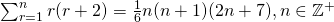When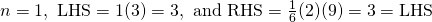is true.

Assume thatis true for some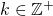, i.e.Want to prove that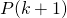is true, i.e.LHS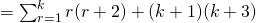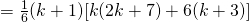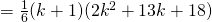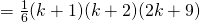Sinceis true andis true, hence by Mathematical Induction,is true for all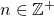(ii)
(a)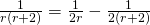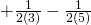(b)
AsHence,is a convergent series, and the value of the sum to infinity isNot readable? Change text.